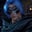Related Tags

java
communitycreator

# How to count the occurrences of each character in JavaGutha Vamsi Krishna

In this shot, we will learn how to count the occurrences of each character in a string using Java.

Let’s begin by defining the problem statement and looking at an example.

### Problem

Write a Java program that will print the number of occurrences of each character in a string.

#### Example

• Input

This is an example

• Output

Occurrences of p = 1

Occurrences of a = 2

Occurrences of s = 2

Occurrences of T = 1

Occurrences of e = 2

Occurrences of h = 1

Occurrences of x = 1

Occurrences of i = 2

Occurrences of l = 1

Occurrences of m = 1

Occurrences of n = 1

### Solution

We will solve this problem using Java HashMap.

• Declare a hashmap that has a key of type Character and value of type Integer.

• Convert string to a character array.

• Traverse every character, and check if it has been added to the hashmap or not.

• If it is has been added to the hashmap, increment the count of that character in hashmap.
• If it has not been added to the hashmap, then save it in the hashmap with the value set as 1.
• Now, print the hashmap.

### Code

import java.io.*;
import java.util.*;

class Solution {
public static void main( String args[] ) {
//given string
String str = "This is an example";

//declare hashmap
HashMap<Character, Integer> count = new HashMap<Character, Integer>();

//convert string to character array
char[] arr = str.toCharArray();

//traverse every character and count the Occurrences
for(char c:arr){

//filter out white spaces
if(c != ' '){
if(count.containsKey(c)){
//if character already traversed, increment it
count.put(c, count.get(c) + 1);
}else{
//if character not traversed, add it to hashmap
count.put(c,1);
}
}

}

//traverse the map and print the number of occurences of a character
for(Map.Entry entry : count.entrySet()){
System.out.println("Occurrences of " + entry.getKey() + " = " + entry.getValue());
}
}
}

### Explanation

In the example above:

• In line 7, the given string str has the value This is an example.

• In line 10, we declare a hashmap count, which has the key of type Character and value of type Integer. It stores the character in key and the count of that character in value.

• In line 13, we convert the string str to character array arr.

• In line 16, we traverse every character in the character array arr.

• In line 19, where we filter out the white spaces in the string, it will check if the present character c is not a white space, then it executes the inner if condition.
• In lines 20 to 25, we check if the present character c is added to the hashmap or not. If it is added, increment the value of that character in the hashmap. If it is not added, then add character c to hashmap with value 1.
• In lines 32 to 34, after traversing every character in arr, hashmap count is filled with occurrences of characters. We then print them one by one.

RELATED TAGS

java
communitycreator

CONTRIBUTORGutha Vamsi Krishna
RELATED COURSES

View all Courses

Keep Exploring

Learn in-demand tech skills in half the time